CBSE Sample Papers for Class 12 Economics Paper 1 are part of CBSE Sample Papers for Class 12 Economics. Here we have given CBSE Sample Papers for Class 12 Economics Paper 1.

## CBSE Sample Papers for Class 12 Economics Paper 1

 Board CBSE Class XII Subject Economics Sample Paper Set Paper 1 Category CBSE Sample Papers

Students who are going to appear for CBSE Class 12 Examinations are advised to practice the CBSE sample papers given here which is designed as per the latest Syllabus and marking scheme as prescribed by the CBSE is given here. Paper 1 of Solved CBSE Sample Paper for Class 12 Economics is given below with free PDF download solutions.

Time : 3 hrs
M.M.: 80

General Instructions

• All questions in both the sections A and B are compulsory. However, there is internal choice in questions of 3,4 and 6 marks.
• Question Nos. 1-4 and 13-16 are very short answer type questions, carrying 1 mark each.
They are required to be answered in one sentence each.
• Question Nos. 5-6 and 17-18 are short answer I type questions, carrying 3 marks each.
Answers to them should not normally exceed 60 words each.
• Question Nos. 7-9 and 19-21 are short answer II type questions, carrying 4 marks each.
Answers to them should not normally exceed 70 words each.
• Question Nos. 10-12 and 22-24 are long answer type questions, carrying 6 marks each.
Answers to them should not normally exceed 100 words each.
• Answers should be brief and to the point and the above word limit be adhered to as far as possible.

Section – A

Question 1.
If 20% increase in price of commodity causes 10% increase in quantity supplied, then elasticity of supply is
(a) elastic
(b) inelastic
(c) perfectly elastic
(d) perfectly inelastic

Question 2.
You are living in a country whose economy is underdeveloped. In this context, why there is a need of efficient utilisation of resources?

Question 3.
The Average Fixed Cost at 4 units of output is ₹ 20. Average variable cost at 5 units of output is 140. Average Cost of producing 5 units is
(a) ₹ 20
(b) ₹ 40
(c) ₹ 56
(d) ₹ 60

Question 4.
Why does variable cost curve originate from the point of origin?

Question 5.
Ice-cream is available at ₹ 10. Akansha who likes ice-cream, has already consumed 4 ice-creams. Her Marginal Utility (MU) of one rupee is 8. Should she consume more ice-cream or should she stop the consumption?

Question 6.
Explain the concept of economics as a positive science and economics as a normative science.
Or
Explain the central problem of ‘choice of technique’?

Question 7.
Show that the average fixed cost curve is a rectangular hyperbola.
Or
Why is the total revenue curve of a competitive firm a straight line passing through origin?

Question 8.
A consumer buys 300 units of good Y at ₹ 15 . The Price Elasticity of Demand for the good is 2. At what price, will he be willing to buy 450 units of good Y?

Question 9.
What factors lead to the emergence of oligopoly market?

Question 10.
From the table given below, determine the level of output at which the producer is in equilibrium. Use the Marginal Revenue (MR) and Marginal Cost (MC) approach. Give reasons for your answer.

 Output (Units) Price (₹) Total Cost (TC) (₹) 1 5 7 2 5 12 3 5 16 4 5 18 5 5 23

Question 11.
Suppose, free entry and exit are allowed in a freely competitive market and there are identical firms in the market. Following are the demand and supply functions of such a market.
Market demand function (qd) = 800 – P
The supply function of a single firm (qs) = 10 + P for P ≥ 20
= 0 for P ≤ 20
Find out the equilibrium price, quantity and number of firms.
Or
Suppose a freely competitive market has identical firms and free entry and exit are also allowed. Market demand function and the supply function of a single firm are given below.
Market demand function (qd) = 590 – P
Market supply function (qs) = 8 + 5P for P ≥ 10 and = 0 for P ≤ 10
(i) What is the significance of P = 10 ?
(ii) At what price will the market be in equilibrium? State the reason.
(iii) Calculate the equilibrium quantity.
(iv) How many firms are required in the market?

Question 12.
Explain the Law of Demand with the help of diagram. State any two of its exceptions. Do you think that law of demand is effective in explaining the demand for gold in our country? Give reason in support of your answer.

Section – B

Question 13.
What is ‘statutory liquidity ratio’?

Question 14.
What is the nature of direct tax?

Question 15.
Explain briefly the meaning of time deposits.

Question 16.
Which of the following is a source of capital receipt?
(a) Foreign donations
(b) Dividends
(c) Disinvestment
(d) Indirect taxes

Question 17.
What do you understand by inflationary gap? Explain with the help of diagram.
Or
Explain the situation of deficient demand in an economy with the help of diagram.

Question 18.
In an economy, total savings are ₹2,000 crore and the ratio of Average Propensity to Save and Average Propensity to Consume is 2 : 7. Calculate the level of income in an economy.

Question 19.
From the following data, calculate net value added at factor cost.

 S.No. Contents ₹ (in lakhs) (i) Sales 500 (ii) Subsidies 30 (iii) Purchase of Machine Installed in the Factory 400 (iv) Purchase of Raw Materials 250 (v) Change in Stock (-)20 (Vi) Consumption of Fixed Capital 40

Question 20.
Explain how do ‘open market operations’ by the Central Bank affect money creation by commercial banks.

Question 21.
Public expenditure is necessary for the development of the country. In the light of the statement, explain any two significance of public expenditure.
Or
Explain any four implications of a large fiscal deficit.

Question 22.
Explain the problem of double counting in the estimation of National Income with the help of an illustration. How can it be avoided?
Or
How will you treat the following while estimating domestic product of India?
(i) Rent received by a resident Indian from his property in Singapore.
(ii) Salaries to Indians working in Japanese Embassy in India.
(iii) Profits earned by a branch of an American Bank in India.
(iv) Salaries paid to Koreans working in Indian Embassy in Korea.

Question 23.
In an economy, aggregate demand is less than aggregate supply. Is the economy in equilibrium? If not, explain the changes that will bring the economy in equilibrium.

Question 24.
Giving reasons explain whether the given statements are true or false.
(i) Current account of balance of payments account records only export and import of goods and services.
(ii) Foreign investments are recorded in the capital account of balance of payments.
(iii) Improvement in exchange rate of the country’s currency always beneficial for Balance of Payment (BoP).
(iv) Rise in foreign exchange rate cause a rise in its supply.

Section – A

(b) inelastic

Developmental needs are more in a underdeveloped economy and these have to be fulfilled with the help of limited resources. Thus, there is a need of efficient and effective utilisation of resources.

(c) ₹ 56
Hint: AFC at 4 units =₹ 20,
∴ TFC = 20 x 4 = ₹ 80
AVC at 5 units = ₹ 40
∴ TVC at 5 units = 40 x 5 = ₹ 200
TC at 5 units = TFC + TVC = 80 + 200 = ₹ 280
ATC = $$\frac { 280 }{ 5 }$$ =₹ 56

Variable cost curve originates from the point of origin because when output is zero, variable cost is also zero.

A consumer attains equilibrium, when = $$\frac { M{ U }_{ X } }{ M{ U }_{ M } } ={ P }_{ X }$$
Where, MUX = Marginal Utility of Commodity X
MUM = Marginal Utility of Money
PX = Price of Commodity X
Substituting the given values, we get $$\frac { M{ U }_{ X } }{ 8 } =10$$ 10 implying that, in a state of equilibrium, Akansha’s MUX must be equal to 80 utils. If having consumed 4th ice-cream, Akansha’s MUX = 80 utils, then she must stop consuming more. However, if MUX > 80, then she should consume more ice-creams till MUX reduces to 80 utils and in terms of its money worth is equal to price of ice cream.

Economics as a Positive Science A positive science is that science in which analysis is confined to cause and effect relationship. It simply states, ’what is’ and not ‘what ought to be1′ It does not pass any value judgement regarding what is right or wrong. Positive economics is concerned with the facts about the economy, as they exist.
e.g. positive economics deals with questions like ’what are the causes of unemployment’, ‘why prices are increasing’ and so on.

Economics as a Normative Science Economics as a normative science is concerned with ’what ought to be’. It examines real economic events from moral and ethical angles and judges whether certain economic events are desirable or not. It passes value judgement and prescribes methods to correct undesirable happenings.
e.g. normative economics deals with opinions like ‘old-age pension should be stopped’, ’MGNREGA will bring an end to unemployment’ and so on.
Or
‘Choice of technique’ is the problem of ’How to produce’?
‘How to produce’? is the problem which refers to the problem relating the choice of technique. Broadly, there are two techniques of production:

1. Labour-intensive technique and
2. capital intensive technique.

Labour-intensive technique uses more of labour than capital (machines) and is cost effective, whereas capital-intensive technique uses more of capital (machines) than labour and promotes efficiency.
So, a business firm needs to choose among these two techniques such that it obtains a cost-effective as well as efficient relationship.

Average Fixed Cost (AFC) can be obtained by dividing Total Fixed Cost (TFC) by the quantity of output (Q),
i. e.

$$AFC=\frac { TFC }{ Q }$$

Since, total fixed costs remain the same as output rises, Average Fixed Cost diminishes but never becomes zero.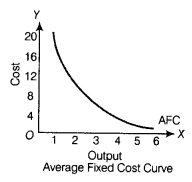As output rises, the Average Fixed Cost (AFC) goes on declining. The AFC curve is, therefore a downward sloping curve. AFC curve never touches either of the axis. Thus, the AFC curve takes the shape of rectangular hyperbola which shows that the area under the curve (i.e. total fixed cost) always remains the same.
Or
A competitive firm sells its output at a uniform price. The price or Average Revenue (AR) is constant and Marginal Revenue (MR) is also constant as it is equal to AR. The Total Revenue (TR) is the sum total of MR corresponding to different levels of output.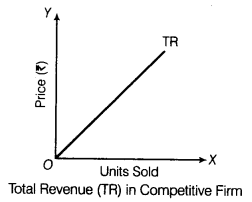Since MR is constant, TR increases at a constant rate. Thus, TR is an upward sloping straight line. It passes through origin because when output is zero, TR is also zero.

Suppose at price X, consumer is willing to buy 450 units of goods Y
Here, Ed = 2;P = 15; P1 = ₹ X, ∆P = ₹ (X-15)
Q = 300 units, Q1 = 450 units .
∆Q = (450 – 300) units = 150 units
Where, Ed = Price Elasticity of Demand
P = Actual Price, P1 = New Price
Q = Actual Quantity, Q1 = New Quantity
∆P = Change in Price,
∆Q = Change in Quantity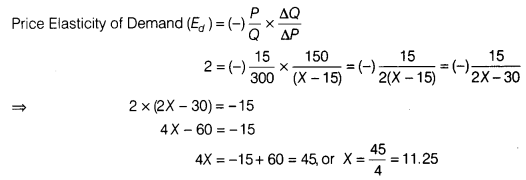The consumer will purchase 450 units of good Y at the price of ₹ 11.25 per unit.

Following factors lead to the emergence of oligopoly market:

1. Government Licensing The government may grant licenses only to a few firms for the production of a commodity, e.g. coal mining rights are given to some companies only.
2. Patent Rights Some firms acquire patent rights on account of their innovative product/ technology that restrict the entry of new firms.
e g. A computerised method of running cash management account, being patented by a company.
3. Scale of Investment Scale of investment for certain products is so high that it becomes difficult for many companies to afford it which leads to concentration of market in the hands of few companies, e.g. Software and Automobile Industries.
4. Cartels Sometimes big companies collaborate or form cartels to gain the control over market.
e.g. OPEC set-up in 1960 by the world’s five major oil producing countries: Iran, Iraq, Kuwait, Saudi Arabia and Venezuela.

Producer’s equilibrium is that point at which a producer is getting maximum profit and he has no tendency to change his output. When Marginal Revenue (MR) is equal to Marginal Cost (MC) and marginal cost is rising beyond the point of equilibrium, at that point, the producer earns maximum profits.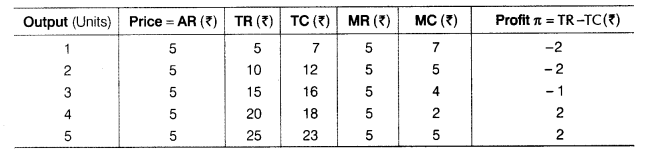Accordingly, producer’s equilibrium is struck at 5th unit of output because at output level of 2 and 5 units, both MR and MC are equal, which is equal to 5 in both the cases. But the producer is in equilibrium at 5th unit only where profit is maximum, i.e. 2. Also, beyond this level, Marginal Cost tends to rise.
Formulae Used
Total Revenue (TR) = Price (P) x Quantity (Q)
Marginal Revenue (MR) = TRN – TRN-1
Marginal Cost (MC) = TCN – TCN-1

From the supply function, we have learnt that the minimum Average Cost (AC) is ₹ 20 and no firm would be ready to sell at price below minimum of AC. Hence, in the situation of free entry and exit, equilibrium price will always be equal to minimum AC. Hence, in this situation, equilibrium price will be P0 = ₹ 20 (equal to minimum AC)

At this price, market supply will be equal to market demand.
From the demand function, we can get the equilibrium quantity by substituting in the function the value of price q0 = 800 – 20 = 780 units

At equilibrium price (P0 = ₹ 20), each firm supplies = 10 + 20 = 30 units
Hence, equilibrium number of firms will be,$$[{ N }_{ 0 }=\frac { { q }_{ 0 } }{ { q }_{ 0p } } =\frac { 780 }{ 30 } =26firm$$

Thus, given the market demand function and supply function of a firm when free entry and exit are allowed,
Equilibrium price = ? 20, Equilibrium quantity = 780 units, Total number of firms = 26
Or

1. P = 10 suggests that in the situation of free entry and exit, equilibrium price can neither be greater nor less than ₹ 10. If the market price becomes greater than ₹ 10, Excess supply emerges and price tends to fall till it becomes equal to ₹ 10. On the other hand, if market price becomes smaller than ₹ 10, excess demand emerges and price tends to rise till it reaches ? 10.
2. From the supply function, $${ q }_{ t }^{ s }$$= 0 for P < 10, we have learnt that the minimum Average Cost (AC) is ₹ 10. In the situation of free entry and exit, equilibrium price is always equal to minimum AC. Hence, in this case, market will be in equilibrium at the price of ₹ 10.
3. From the demand function, we can get the equilibrium quantity by substituting the value of price.
q0 = 590 – P = 590 -10 = 580 units
4. At equilibrium price (P0) = 10, each firm supplies q0f = 8+ 5 x 10 = 58 units
Hence, the number of firms required =$$\frac { 580 }{ 58 } =10firms$$

The Law of Demand states that other things remaining constant, the quantity demanded of a commodity expands with fall in its price and contracts with a rise in its price. So, there is an inverse relationship between price and quantity demanded of a commodity. This is explained with the help of an imaginary schedule and cunve which is based on that imaginary schedule.

 Price per unit (₹) Quantity Demanded (units) 10 50 8 60 6 70 4 80 2 90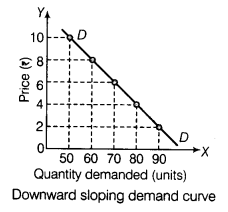Exceptions to the Law The law will not hold good under following circumstances:

1. Conspicuous Consumption The Law of Demand will not apply in case of costly items such as diamonds. These commodities will be demanded, even if the prices have gone very high.
2. Ignorance If the consumer is not aware of the competitive price of the commodity, he may purchase
more of the commodity even at higher price. Such ignorance of the buyers makes the Law of Demand ineffective.

No, I don’t think that law of demand explains the demand for gold in our country. In spite of continuously rising prices, demand for gold remains constant. It is because consumers believe that prices of gold will rise further in future and because of this their demand has not fallen in the present. In this case too, law of demand have failed due to high future expectation of price by the consumer.

Section – B

Fraction of total deposit that each commercial bank must keep with themselves in liquid assets (cash, gold and unencumbered securities) is referred to as statutory liquidity ratio.

Direct tax is that tax whose incidence and impact falls on the same person i.e. burden of such taxes cannot be shifted to others e.g. gift tax, corporation tax, income tax, etc.

Time deposits are fixed or recurring deposits which have a fixed period of maturity. The term of deposit may vary. Cheques cannot be issued against them and they are not payable on demand but these deposits yield interest for the depositor.

(c) Disinvestment is a source of capital receipt.

The extent to which current aggregate demand becomes higher than the aggregate demand required for full employment, is termed as inflationary gap.

In the figure given below, full employment equilibrium is struck at point E. If the level of demand increases to AD,, it is in excess of what is required to maintain full employment. This causes inflation.Or

The extent to which aggregate demand falls short of aggregate supply to maintain full employment equilibrium is known as deficient demand. As shown in the diagram, deficient demand occurs when Aggregate Demand (AD) is less than what is required to maintain full employment in the economy. Full employment level of demand is indicated by AD1. If demand level happens to be AD2 the gap between AD1 and AD2, equal to ab, represents deficient demand.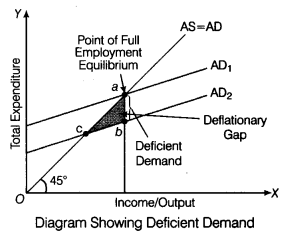Let Average Propensity to Save (APS) = 2x
Average Propensity to Consume (APC) = 7x
Now, APS + APC = 1
2x + 7x = 1
9x = 1
$$x=\frac { 1 }{ 9 }$$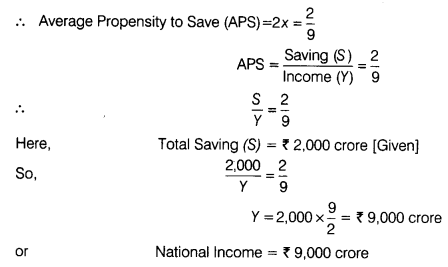Net Value Added at Factor Cost (NVAFC) = Sales + Change in Stock – Purchase of Raw Materials – Consumption of Fixed Capital + Subsidies =
500 + (-20) – 250 – 40 + 30
= 530 – 310 = ₹ 220 lakhs

Under open market operations, RBI purchases or sells government securities to general public for the purpose of increasing or decreasing the stock of money in an economy. The purchase or sale of securities controls the money in the hands of public as they deposit or withdraw the money from commercial banks. Thus, money creation by commercial banks get affected.

Suppose, the Central Bank purchase securities of ₹ 1,000 from a bond holder by issuing a cheque. The seller of the bond produces this cheque of ₹ 1,000 to his commercial bank, fhe commercial bank credits the account of the seller by ₹ 1,000 and the deposits of the bank goes up by ₹ 1,000, which is a liability for the bank. On the other hand, the assets of the commercial bank also goes up as the cheque in its possession is a claim on the Central Bank.

Thus, purchase of security increases the money creation of commercial banks and similarly sale of securities decreases the credit creation of commercial banks. Thus, the Central Bank controls the process of money creation by commercial banks through open market operations.

The two significance of public expenditure are as follows:

1. Increases Economic Growth Public expenditure accelerates the process of economic growth. This is of unique importance particularly, in the context of developing countries like India. Public expenditure helps in establishing new industries. It ensures development of public overhead cost like the construction of roads, dams, bridges, etc.
2. Increases Economic Welfare Public expenditure increases economic welfare. This happens particularly when public expenditure is directed towards poverty eradication, as well as, health and education for the poorer sections of the society. Such expenditures reduce economic divide between ‘have’ and ‘have-nots’ and thereby promotes social welfare.

Or
Following are the implications of large fiscal deficit:

1. National Debt Fiscal deficit leads to national debt as government resorts to borrowings to combat the deficit. National debt is a burden on future generations. Therefore, future generations inherit a economy which is under high pressure of mounting borrowings.
2. Low GDP Growth In case of large fiscal deficit, a significant percentage of national income is used up to pay the’past debts and very less is spent on infrastructural expansion, therefore, GDP growth of the economy remains low.
3. Crowding-out Effect High fiscal deficit increases borrowings by the government from the money market which reduces availability of funds for private entrepreneurs. Shortage of funds leads to high rate of interest which lowers the level of investment in the economy. Thus, economy slips into state of economic slowdown. This is known as crowding-out effect.
4. Erosion of Government Credibility High fiscal deficit erodes credibility of the government in domestic as well as international market, ‘credit rating’ of the government is lowered and global investors start withdrawing their investment from domestic economy.

The counting of the value of commodity more than once is called double counting. This leads to over estimation of the value of goods and services produced. Thus, the importance of avoiding double counting lies in correct estimation of the value of domestic product, e.g. a farmer produces one tonne of wheat and sells it for ₹ 400 in the market to a flour mill.

The flour mill sells it for ₹ 600 to the baker. The baker sells the bread to the shopkeeper for ₹ 800. The shopkeeper sells the entire bread to the final consumers for ₹ 900. Thus,
Value of Output = ₹ (400 + 600 + 800 + 900) = ₹ 2,700

In fact, the value of the wheat is counted four times, the value of services of the miller thrice and the value of services by the baker twice.
In other words, the value of wheat and value of services of the miller and of the baker have been counted more than once. The counting of the value of commodity more than once is called double counting. To avoid the problem of double counting, following two methods are used:

1. Final Output Method According to this method, the value of intermediate goods is not considered. Only the value of final goods and services is considered. In the above example, the value of final goods, i.e. bread is ? 900.
2. Value Added Method Another method to avoid the problem of double counting is to estimate the total value added at each stage of production. In the above example, the value added at each stage of production is ₹ (400 + 200 + 200 +100)= ₹ 900.

Or

1. It will not be included in domestic product of India as this income is earned outside the domestic (economic) territory of India.
2. It will not be a part of domestic product of India as embassy of Japan in India is not a part of domestic territory of India. Hence, this income is not earned within the domestic territory of India.
3. It will be included in domestic product of India as the branch of American bank is located within the domestic territory of India. So, it is income earned within the domestic territory of India.
4. It will be part of domestic product of India because this income is earned within the domestic territory of India. Indian Embassy in Korea is treated as located within the domestic territory of India.

If Aggregate Demand (AD) is less than Aggregate Supply (AS), then economy is not in equilibrium because an economy is in equilibrium when AS = AD.
When AD < AS, flow of goods and services in the economy tends to exceed their demand. As a result, some of the goods would remain unsold.
To clear unwanted stocks, the producers would plan a cut in production. Consequently, AS would reduce to become equal to AD. This is how AS adapts itself to AD.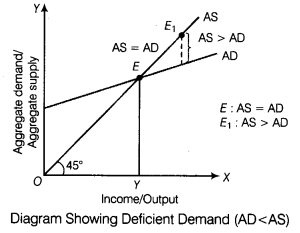In the above figure, equilibrium is struck at point E
where, AD = AS. At point Ev Aggregate Supply exceeds Aggregate Demand (AS > AD).
It will cause following changes in the economy:

1. Stocks of the producers would be in excess of the desired limit.
2. Desired level of output for the subsequent year will face a cut and profits will start shrinking.
3. Levels of income and employment will tend to shrink to the point where, S = l, corresponding to point E in the diagram.
Thus, the economy will come back to the state of equilibrium.